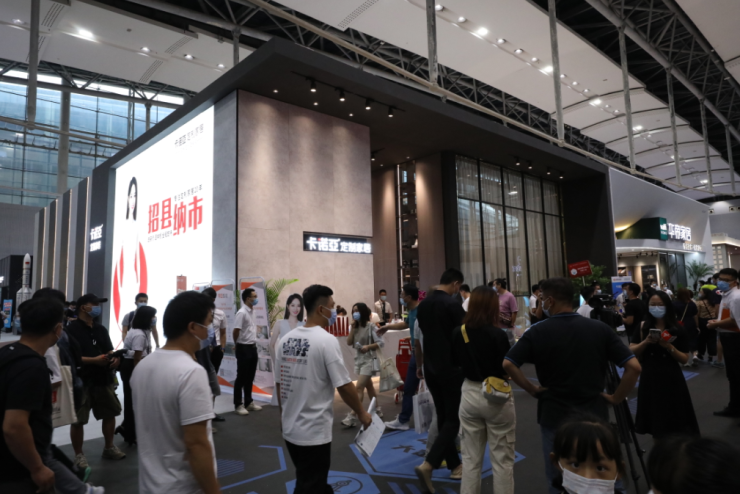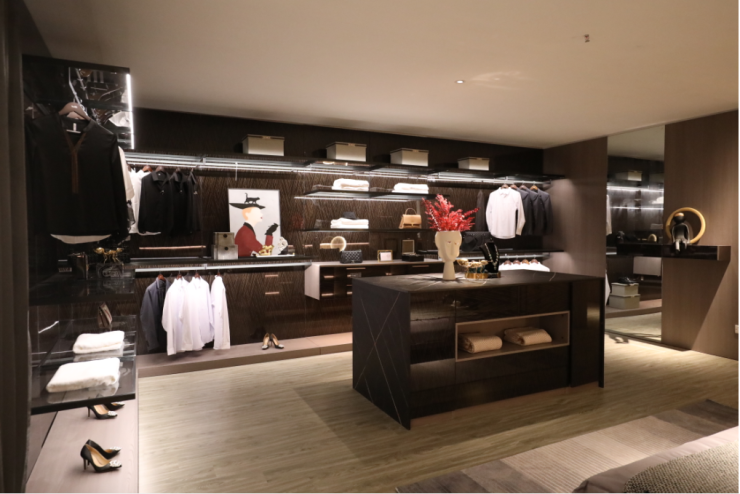|

# 卡诺亚李伟明:以高颜值与高品质的产品,打造一站式定制服务

2021年7月20日—7月23日，第二十三届中国建博会（广州）在广州广交会展馆、保利世贸博览馆盛大开幕。中国建博会（广州）作为大家居建装行业的一场盛会，集结产业链上下游2000家+参展企业，吸引来自国内外的社会各界人士前来参观体验。【搜狐焦点家居】：每一年的广州建博会，向来是冠军企业的首秀平台，今年，贵司带来了哪些新产品？都有哪些新看点与新趋势？

【李伟明】：2021年的广州建博会，卡诺亚定制家居带来了最新研发的产品：高端产品睿系列和馨晴时光系列、米兰之吻系列，3大新品看点还是很多的：

【搜狐焦点家居】：这一届的年轻消费群体都追求新潮生活，那他们对家居的核心消费诉求是什么？换言之达成“新潮生活”应该具备哪些要素？贵司又是如何拉近与消费者的距离的呢？

【李伟明】：随着80后、90后为主的新中产消费群的崛起，他们对生活品质的要求逐渐提高，追求新潮生活，对家具消费方面的关注点逐渐从产品性价比、实用性等方面，向产品外观设计、功能设计等方面转移，追求“颜价比”。对于定制家居行业而言，为年轻消费群打造“新潮生活”，必须具备产品个性化、体验场景化、服务专业化、品牌年轻化、沟通娱乐化这5大要素。【搜狐焦点家居】：精装修时代，传统家装已经被工程取代，随着消费者对一站式服务的需求升级，整装在2021年再次爆发成为风口。请问您是如何看待整装风潮的呢？贵司在整装上是否有进一步的探索？

【李伟明】：无论是拓展客户资源的新渠道，还是推进品牌成长的新赛道，高达五万亿的整装市场大蛋糕，对于定制家居企业都具有很大诱惑力。可以说，整装一定是未来家装消费的一大趋势。

【搜狐焦点家居】：过去一年“数字化”可谓行业热词，家居数字化转型势在必行，那您认为家居企业的数字化升级体现在哪些方面？贵司接下来在数字化升级战略中有哪些具体的规划？

【李伟明】：家居企业的数字化升级，应该体现在企业经营的方方面面，包括研发、设计、销售、生产、营销、终端、交付、服务等全链路。卡诺亚接下来在数字化升级战略中，将紧紧围绕“数字赋能，品牌制胜”的年度战略方针展开，从生产管理ERP系统，到客户管理CRM系统，将在厂商内部全面推行营销体系的数字化升级，有效管理专卖店运营各环节，把复杂的定制运营管理，变成简单高效的标准流程，让繁杂的定制流程变得更加简单。近期我们将聚焦数字化专卖店建设， 大力推广卡诺亚数字门店，经销商可通过线上专卖店跟进管理客户，从而提升客户沟通效率、转化率。我们的数字化转型，不仅包含了数字化工具和系统，还包含了每个员工和每个经销商使用数字化的能力。

【搜狐焦点家居】：除了数字化，“高定热”吸引了许多其他企业进入这个细分领域，高定行业发展如此火热，可否分析下它的发展逻辑与未来趋势？入局高定者，又该如何打造出品牌的差异化？

【李伟明】：数据显示，新中产阶级对高品质产品的追求，导致当前”高定热“的形成。“高定”是刚刚启航的新赛道，放眼未来，相信“高定”赛道将会给一些具有更高的品质意识与服务意识的品牌制造更多的机会。现阶段的“高定”，更多是通过设计和材质上的高标准、高价值去输出，但未来还是要落实到服务和品牌的价值上面去，这也是未来入局“高定”这条新赛道，真正能做出品牌差异化的重要手段。【搜狐焦点家居】：随着地产红利的逐渐消失，建材家居行业增速放缓，如何通过营销破局，获取新增长成为关键，贵司今年在营销战略方面有哪些重大突破呢？

【李伟明】：卡诺亚今年在营销战略层面的突破，将紧紧围绕年度战略方针展开：

①加大品牌的宣传。2021年，卡诺亚加大品牌的宣传，在中国之声、音乐之声等媒体投入更多的宣传。

②品牌的年轻化。卡诺亚在打造品牌年轻化时，签约明星蓝盈莹只是迈出的第一步。今后将更加充分挖掘年轻群体对于家居生活最为核心的价值诉求点和情感认同点，塑造年轻化品牌形象。

③沟通更多样。如今的年轻消费者，接受网络信息变得碎片化和多元化，所以，卡诺亚与消费者的沟通也呈现多样化，注重口碑内容营销，让卡诺亚家喻户晓。在2021年里，公司加大品牌宣传，以及与消费者沟通形式的多样化，增加了许多的互联网种草的渠道，在知乎、小红书、一兜糖、好好住等平台加大内容的输出，便于消费者更好地接触到卡诺亚。

④数字化赋能。对于门店来说，如今引流获客成本高、成交难度越来越大。为了解决这一痛点，卡诺亚大力推广数字门店，帮助终端门店做好数字化营销内容，实现低成本引流，提高客单值，提升成交率。

【搜狐焦点家居】：您认为广州建博会对参展企业的重要意义体现在哪些方面？今年的建博会给您最大的感受是什么？

【李伟明】：广州建博会作为行业第一盛会，为参展企业提供了一个很好的展示交流平台，为行业同仁们了解新技术、新产品、新材料提供了便利，卡诺亚很高兴借助这个平台认识了更多的志同道合的朋友。

`声明：本文由入驻焦点开放平台的作者撰写，除焦点官方账号外，观点仅代表作者本人，不代表焦点立场错误信息举报电话： 400-099-0099，邮箱：jubao@vip.sohu.com，或点此进行意见反馈，或点此进行举报投诉。`A B C D E F G H J K L M N P Q R S T W X Y Z
A - B - C - D - E
• A
• 鞍山
• 安庆
• 安阳
• 安顺
• 安康
• 澳门
• B
• 北京
• 保定
• 包头
• 巴彦淖尔
• 本溪
• 蚌埠
• 亳州
• 滨州
• 北海
• 百色
• 巴中
• 毕节
• 保山
• 宝鸡
• 白银
• 巴州
• C
• 承德
• 沧州
• 长治
• 赤峰
• 朝阳
• 长春
• 常州
• 滁州
• 池州
• 长沙
• 常德
• 郴州
• 潮州
• 崇左
• 重庆
• 成都
• 楚雄
• 昌都
• 慈溪
• 常熟
• D
• 大同
• 大连
• 丹东
• 大庆
• 东营
• 德州
• 东莞
• 德阳
• 达州
• 大理
• 德宏
• 定西
• 儋州
• 东平
• E
• 鄂尔多斯
• 鄂州
• 恩施
F - G - H - I - J
• F
• 抚顺
• 阜新
• 阜阳
• 福州
• 抚州
• 佛山
• 防城港
• G
• 赣州
• 广州
• 桂林
• 贵港
• 广元
• 广安
• 贵阳
• 固原
• H
• 邯郸
• 衡水
• 呼和浩特
• 呼伦贝尔
• 葫芦岛
• 哈尔滨
• 黑河
• 淮安
• 杭州
• 湖州
• 合肥
• 淮南
• 淮北
• 黄山
• 菏泽
• 鹤壁
• 黄石
• 黄冈
• 衡阳
• 怀化
• 惠州
• 河源
• 贺州
• 河池
• 海口
• 红河
• 汉中
• 海东
• 怀来
• I
• J
• 晋中
• 锦州
• 吉林
• 鸡西
• 佳木斯
• 嘉兴
• 金华
• 景德镇
• 九江
• 吉安
• 济南
• 济宁
• 焦作
• 荆门
• 荆州
• 江门
• 揭阳
• 金昌
• 酒泉
• 嘉峪关
K - L - M - N - P
• K
• 开封
• 昆明
• 昆山
• L
• 廊坊
• 临汾
• 辽阳
• 连云港
• 丽水
• 六安
• 龙岩
• 莱芜
• 临沂
• 聊城
• 洛阳
• 漯河
• 娄底
• 柳州
• 来宾
• 泸州
• 乐山
• 六盘水
• 丽江
• 临沧
• 拉萨
• 林芝
• 兰州
• 陇南
• M
• 牡丹江
• 马鞍山
• 茂名
• 梅州
• 绵阳
• 眉山
• N
• 南京
• 南通
• 宁波
• 南平
• 宁德
• 南昌
• 南阳
• 南宁
• 内江
• 南充
• P
• 盘锦
• 莆田
• 平顶山
• 濮阳
• 攀枝花
• 普洱
• 平凉
Q - R - S - T - W
• Q
• 秦皇岛
• 齐齐哈尔
• 衢州
• 泉州
• 青岛
• 清远
• 钦州
• 黔南
• 曲靖
• 庆阳
• R
• 日照
• 日喀则
• S
• 石家庄
• 沈阳
• 双鸭山
• 绥化
• 上海
• 苏州
• 宿迁
• 绍兴
• 宿州
• 三明
• 上饶
• 三门峡
• 商丘
• 十堰
• 随州
• 邵阳
• 韶关
• 深圳
• 汕头
• 汕尾
• 三亚
• 三沙
• 遂宁
• 山南
• 商洛
• 石嘴山
• T
• 天津
• 唐山
• 太原
• 通辽
• 铁岭
• 泰州
• 台州
• 铜陵
• 泰安
• 铜仁
• 铜川
• 天水
• 天门
• W
• 乌海
• 乌兰察布
• 无锡
• 温州
• 芜湖
• 潍坊
• 威海
• 武汉
• 梧州
• 渭南
• 武威
• 吴忠
• 乌鲁木齐
X - Y - Z
• X
• 邢台
• 徐州
• 宣城
• 厦门
• 新乡
• 许昌
• 信阳
• 襄阳
• 孝感
• 咸宁
• 湘潭
• 湘西
• 西双版纳
• 西安
• 咸阳
• 西宁
• 仙桃
• 西昌
• Y
• 运城
• 营口
• 盐城
• 扬州
• 鹰潭
• 宜春
• 烟台
• 宜昌
• 岳阳
• 益阳
• 永州
• 阳江
• 云浮
• 玉林
• 宜宾
• 雅安
• 玉溪
• 延安
• 榆林
• 银川
• Z
• 张家口
• 镇江
• 舟山
• 漳州
• 淄博
• 枣庄
• 郑州
• 周口
• 驻马店
• 株洲
• 张家界
• 珠海
• 湛江
• 肇庆
• 中山
• 自贡
• 资阳
• 遵义
• 昭通
• 张掖
• 中卫

1室1厅1厨1卫1阳台

1
2
3
4
5

0
1
2

1

1

0
1
2
3报名成功，资料已提交审核A B C D E F G H J K L M N P Q R S T W X Y Z
A - B - C - D - E
• A
• 鞍山
• 安庆
• 安阳
• 安顺
• 安康
• 澳门
• B
• 北京
• 保定
• 包头
• 巴彦淖尔
• 本溪
• 蚌埠
• 亳州
• 滨州
• 北海
• 百色
• 巴中
• 毕节
• 保山
• 宝鸡
• 白银
• 巴州
• C
• 承德
• 沧州
• 长治
• 赤峰
• 朝阳
• 长春
• 常州
• 滁州
• 池州
• 长沙
• 常德
• 郴州
• 潮州
• 崇左
• 重庆
• 成都
• 楚雄
• 昌都
• 慈溪
• 常熟
• D
• 大同
• 大连
• 丹东
• 大庆
• 东营
• 德州
• 东莞
• 德阳
• 达州
• 大理
• 德宏
• 定西
• 儋州
• 东平
• E
• 鄂尔多斯
• 鄂州
• 恩施
F - G - H - I - J
• F
• 抚顺
• 阜新
• 阜阳
• 福州
• 抚州
• 佛山
• 防城港
• G
• 赣州
• 广州
• 桂林
• 贵港
• 广元
• 广安
• 贵阳
• 固原
• H
• 邯郸
• 衡水
• 呼和浩特
• 呼伦贝尔
• 葫芦岛
• 哈尔滨
• 黑河
• 淮安
• 杭州
• 湖州
• 合肥
• 淮南
• 淮北
• 黄山
• 菏泽
• 鹤壁
• 黄石
• 黄冈
• 衡阳
• 怀化
• 惠州
• 河源
• 贺州
• 河池
• 海口
• 红河
• 汉中
• 海东
• 怀来
• I
• J
• 晋中
• 锦州
• 吉林
• 鸡西
• 佳木斯
• 嘉兴
• 金华
• 景德镇
• 九江
• 吉安
• 济南
• 济宁
• 焦作
• 荆门
• 荆州
• 江门
• 揭阳
• 金昌
• 酒泉
• 嘉峪关
K - L - M - N - P
• K
• 开封
• 昆明
• 昆山
• L
• 廊坊
• 临汾
• 辽阳
• 连云港
• 丽水
• 六安
• 龙岩
• 莱芜
• 临沂
• 聊城
• 洛阳
• 漯河
• 娄底
• 柳州
• 来宾
• 泸州
• 乐山
• 六盘水
• 丽江
• 临沧
• 拉萨
• 林芝
• 兰州
• 陇南
• M
• 牡丹江
• 马鞍山
• 茂名
• 梅州
• 绵阳
• 眉山
• N
• 南京
• 南通
• 宁波
• 南平
• 宁德
• 南昌
• 南阳
• 南宁
• 内江
• 南充
• P
• 盘锦
• 莆田
• 平顶山
• 濮阳
• 攀枝花
• 普洱
• 平凉
Q - R - S - T - W
• Q
• 秦皇岛
• 齐齐哈尔
• 衢州
• 泉州
• 青岛
• 清远
• 钦州
• 黔南
• 曲靖
• 庆阳
• R
• 日照
• 日喀则
• S
• 石家庄
• 沈阳
• 双鸭山
• 绥化
• 上海
• 苏州
• 宿迁
• 绍兴
• 宿州
• 三明
• 上饶
• 三门峡
• 商丘
• 十堰
• 随州
• 邵阳
• 韶关
• 深圳
• 汕头
• 汕尾
• 三亚
• 三沙
• 遂宁
• 山南
• 商洛
• 石嘴山
• T
• 天津
• 唐山
• 太原
• 通辽
• 铁岭
• 泰州
• 台州
• 铜陵
• 泰安
• 铜仁
• 铜川
• 天水
• 天门
• W
• 乌海
• 乌兰察布
• 无锡
• 温州
• 芜湖
• 潍坊
• 威海
• 武汉
• 梧州
• 渭南
• 武威
• 吴忠
• 乌鲁木齐
X - Y - Z
• X
• 邢台
• 徐州
• 宣城
• 厦门
• 新乡
• 许昌
• 信阳
• 襄阳
• 孝感
• 咸宁
• 湘潭
• 湘西
• 西双版纳
• 西安
• 咸阳
• 西宁
• 仙桃
• 西昌
• Y
• 运城
• 营口
• 盐城
• 扬州
• 鹰潭
• 宜春
• 烟台
• 宜昌
• 岳阳
• 益阳
• 永州
• 阳江
• 云浮
• 玉林
• 宜宾
• 雅安
• 玉溪
• 延安
• 榆林
• 银川
• Z
• 张家口
• 镇江
• 舟山
• 漳州
• 淄博
• 枣庄
• 郑州
• 周口
• 驻马店
• 株洲
• 张家界
• 珠海
• 湛江
• 肇庆
• 中山
• 自贡
• 资阳
• 遵义
• 昭通
• 张掖
• 中卫• 手机• 分享
• 设计
免费设计
• 计算器
装修计算器
• 联系
• 置顶
返回顶部